This topic describes how to use sort keys to accelerate queries in AnalyticDB for PostgreSQL.

## Background information

When you create a table, you can specify one or more columns as the sort key. After data is written to the table, you can sort the table data by sort key.

Sorting accelerates range queries. After sorting, the maximum and minimum values for each column are recorded to accelerate range queries. If a query uses range conditions in the WHERE clause, the query engine of AnalyticDB for PostgreSQL can use the maximum and minimum values to skip blocks out of the specific range during table scans.

For example, assume that a table stores seven years of data sorted by date. If you want to query the data of a specific month, only 1/(7 × 12) of the table data needs to be scanned and 98.8% of the disk blocks can be skipped during the scan. If the data is not sorted by date, all disk blocks may be scanned.

AnalyticDB for PostgreSQL supports two sorting methods:

• Compound sorting: applies to scenarios where the filter condition is a prefix subset of the sort key. For example, this method can be used if the filter condition of an SQL statement includes the first column of the sort key.
• Interleaved sorting: allocates an equal weight to each column in the sort key. This sorting method is more useful for scenarios where the query condition includes a filter condition subset.

## Select a sort key

• If specific columns are frequently used in the equivalence or range query condition of your SQL queries, you can use these columns as sort keys. This accelerates the SQL queries by using data sorting and rough set indexes. In most cases, we recommend that you use compound sorting.
• If the columns contained in filter conditions vary from query to query, you can use interleaved sorting to accelerate queries. In most cases, interleaved sorting takes longer than compound sorting. This is because interleaved sorting requires extra analysis to be performed on the data.
Note An interleaved sort key can contain up to eight columns.
• If you frequently use a specific column as the JOIN condition, you can specify the JOIN column as both the distribution key and the sort key. This allows you to choose a merge join instead of a hash join. The sort phase of the merge join can be skipped because the data is already sorted based on the join key.

## Performance comparison between compound sorting and interleaved sorting

In this section, two tables that contain the same data are separately sorted by using compound sorting and interleaved sorting. The results of queries on the tables show that the two sorting methods provide different performance in different scenarios.

1. Execute the following statements to create two tables that have the id, num1, num2, and value columns, and specify the id, num1, and num2 columns as the sort key of the two tables:
``````CREATE TABLE test(id int, num1 int, num2 int, value varchar)
with(APPENDONLY=TRUE, ORIENTATION=column)
DISTRIBUTED BY(id)
ORDER BY(id, num1, num2);

CREATE TABLE test_multi(id int, num1 int, num2 int, value varchar)
with(APPENDONLY=TRUE, ORIENTATION=column)
DISTRIBUTED BY(id)
ORDER BY(id, num1, num2);``````
2. Execute the following statements to insert 10 millions rows of data to the two tables:
``````INSERT INTO test(id, num1, num2, value) select g,
(random()*10000000)::int,
(random()*10000000)::int,
(array['foo', 'bar', 'baz', 'quux', 'boy', 'girl', 'mouse', 'chlid', 'phone'])[floor(random() * 10 +1)]
FROM generate_series(1, 10000000) as g;

INSERT INTO test_multi SELECT * FROM test;``````

Execute the following statements to query the total number of rows in the two tables:

• ``SELECT count(*) FROM test;``

The following information is returned:

``````  count
----------
10000000
(1 row)``````
• ``SELECT count(*) FROM test_multi;``

The following information is returned:

``````  count
----------
10000000
(1 row)``````
3. Separately sort the two tables by using compound sorting and interleaved sorting.

Execute the following statement to sort the test table by using compound sorting:

``SORT test;``

Execute the following statement to sort the test_multi table by using interleaved sorting:

``MULTISORT test_multi;``
4. Compare the equivalence query performance.
• Q1 is filtered based on the first column of the sort key.
``````SELECT * FROM test WHERE id = 100000;
SELECT * FROM test_multi WHERE id = 100000;``````
• Q2 is filtered based on the second column of the sort key.
``````SELECT * FROM test WHERE num1 = 8766963;
SELECT * FROM test_multi WHERE num1 = 8766963;``````
• Q3 is filtered based on the second and third columns of the sort key.
``````SELECT * FROM test WHERE num1 = 100000 AND num2=2904114;
SELECT * FROM test_multi WHERE num1 = 100000 AND num2=2904114;``````

The following table describes the performance comparison results.

Sorting method Q1 Q2 Q3
Compound sorting 0.026s 3.95s 4.21s
Interleaved sorting 0.55s 0.42s 0.071s
5. Compare the range query performance.
• Q1 is filtered based on the first column of the sort key.
``````SELECT count(*) FROM test WHERE id>5000 AND id < 100000;
SELECT count(*) FROM test_multi WHERE id>5000 AND id < 100000;``````
• Q2 is filtered based on the second column of the sort key.
``````SELECT count(*) FROM test WHERE num1 >5000 AND num1 <100000;
SELECT count(*) FROM test_multi WHERE num1 >5000 AND num1 <100000;``````
• Q3 is filtered based on the second and third columns of the sort key.
``````SELECT count(*) FROM test WHERE num1 >5000 AND num1 <100000 AND num2 < 100000;
SELECT count(*) FROM test_multi WHERE num1 >5000 AND num1 <100000 AND num2 < 100000;``````

The following table describes the performance comparison results.

Sorting method Q1 Q2 Q3
Compound sorting 0.07s 3.35s 3.64s
Interleaved sorting 0.44s 0.28s 0.047s

Test conclusions:

• Q1 uses the first column of the sort key to filter data. In this case, queries that use compound sorting have a shorter response time than those that use interleaved sorting.
• Q2 uses a non-first column of the sort key to filter data. In this case, queries that use interleaved sorting perform significantly better than those that use compound sorting.
• Q3 uses non-first columns of the sort key to filter data. In this case, queries that use interleaved sorting are faster and more effective than those that use compound sorting. The more columns of the interleaved sort key used, the better the performance of the queries that use interleaved sorting.

## Sorting acceleration

The sorting acceleration feature is supported. After you execute a `SORT <tablename>` statement, the system sorts the data of the specified table. Then, AnalyticDB for PostgreSQL pushes operators such as SORT down to the storage layer so that queries are accelerated based on the physical order of data. When your underlying data is ordered, your queries can be accelerated. This feature can accelerate queries that contain SORT, AGG, or JOIN operators based on sort keys.

Note
• The sorting acceleration feature requires all of your data to be ordered. After you write data, you must execute the `SORT <tablename>` statement again to sort data.
• By default, the sorting acceleration feature is enabled.

In this example, a test table named far is used to compare the query time before and after sorting acceleration.

1. Execute the following statement to create a test table named far:
``````CREATE TABLE far(a int,  b int)
WITH (APPENDONLY=TRUE, COMPRESSTYPE=ZSTD, COMPRESSLEVEL=5)
DISTRIBUTED BY (a)  --Distribution key
ORDER BY (a);       --Sort key``````
2. Execute the following statement to write one million rows of data to the far table:
``INSERT INTO far VALUES(generate_series(0, 1000000), 1);``
3. Execute the following statement to sort the data in the far table:
``SORT far;``

Query performance comparison:

Note The query time results in this example are for reference only. The query time varies based on various factors such as the data volume, computing resources, and network conditions.
• ORDER BY
• Before sorting acceleration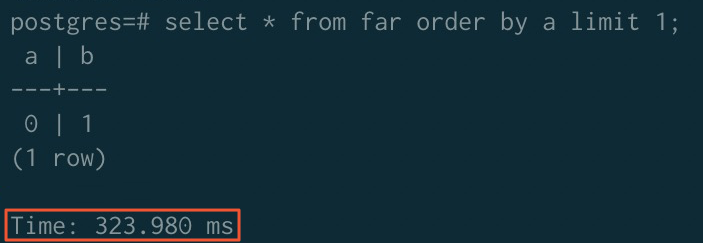• After sorting acceleration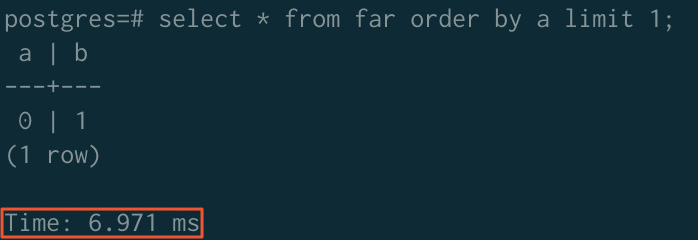• GROUP BY
• Before sorting acceleration• After sorting acceleration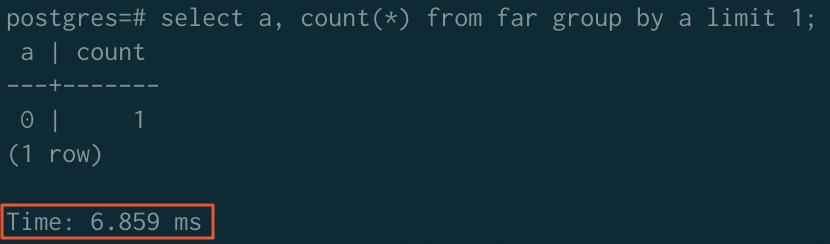• JOIN
• Before sorting acceleration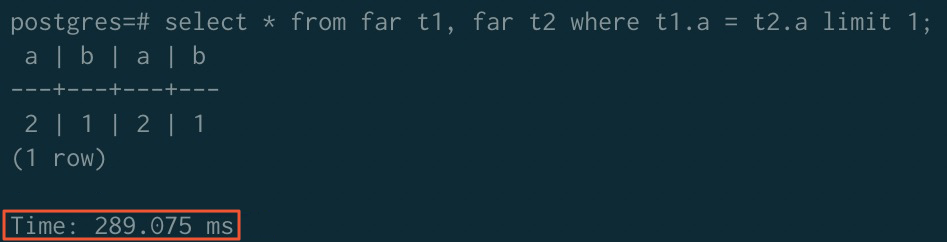• After sorting acceleration
Note If you want to use the sorting acceleration feature for JOIN operators, you must execute the following statements to disable the ORCA optimizer and enable the merge join algorithm:
``````SET enable_mergejoin TO on;
SET optimizer TO off;``````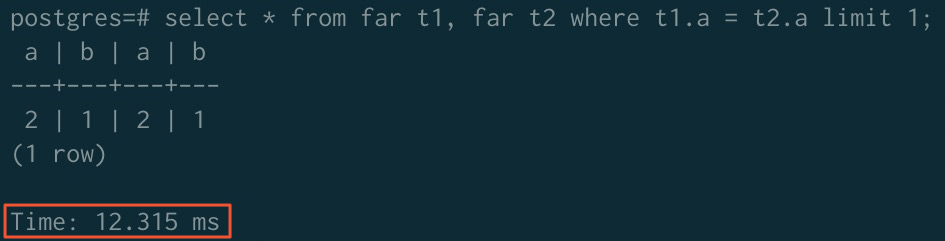- ORDER BY GROUP BY JOIN
Before acceleration 323.980 ms 779.368 ms 289.075 ms
After acceleration 6.971 ms 6.859 ms 12.315 ms